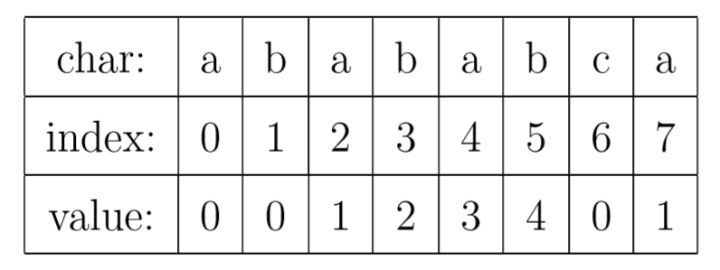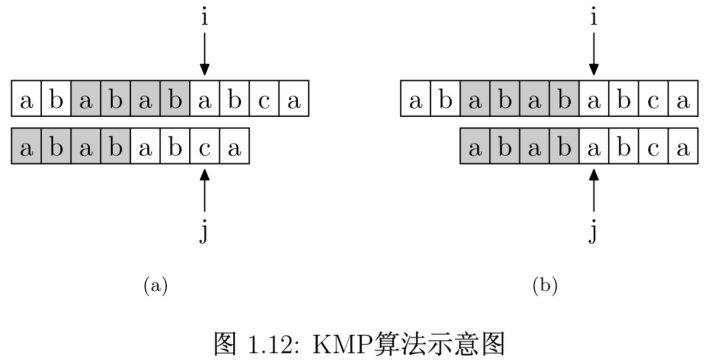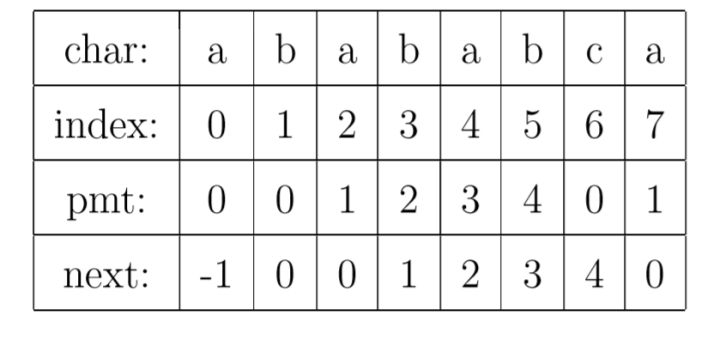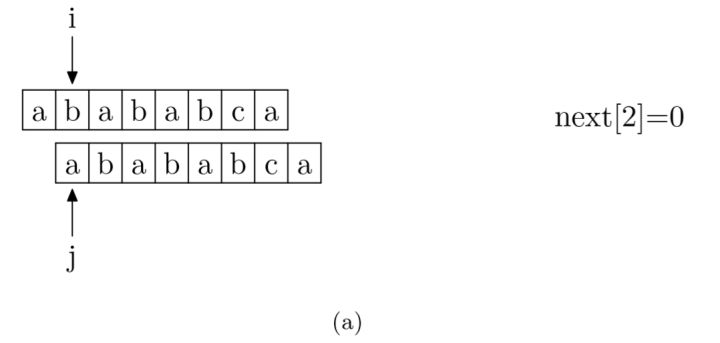# KMP 算法

## 定义

Knuth-Morris-Pratt 字符串查找算法，简称为 “KMP算法”，常用于在一个文本串S内查找一个模式串P 的出现位置，这个算法由Donald Knuth、Vaughan Pratt、James H. Morris三人于1977年联合发表，故取这3人的姓氏命名此算法。

## 算法流程

1. 如果j = -1，或者当前字符匹配成功（即S[i] == P[j]），都令i++，j++，继续匹配下一个字符；

2. 如果j != -1，且当前字符匹配失败（即S[i] != P[j]），则令 i 不变，j = next[j]。此举意味着失配时，模式串P相对于文本串S向右移动了j - next [j] 位。

3. 换言之，当匹配失败时，模式串向右移动的位数为：失配字符所在位置 - 失配字符对应的next 值（next 数组的求解会在下文的3.3.3节中详细阐述），即移动的实际位数为：j - next[j]，且此值大于等于1。


# PMT 是什么

KMP算法的核心，是一个被称为部分匹配表(Partial Match Table)的数组。

## PMT 简介# 如何加速查找## C 语言实现

int KMP(char * t, char * p)
{
int i = 0;
int j = 0;

while (i < strlen(t) && j < strlen(p))
{
if (j == -1 || t[i] == p[j])
{
i++;
j++;
}
else
j = next[j];
}

if (j == strlen(p))
return i - j;
else
return -1;
}## 获取 next 数组代码

void getNext(char * p, int * next)
{
next = -1;
int i = 0, j = -1;

while (i < strlen(p))
{
if (j == -1 || p[i] == p[j])
{
++i;
++j;
next[i] = j;
}
else
j = next[j];
}
}


# BM算法

KMP的匹配是从模式串的开头开始匹配的，而1977年，德克萨斯大学的Robert S. Boyer教授和J Strother Moore教授发明了一种新的字符串匹配算法：

Boyer-Moore算法，简称BM算法。

BM算法定义了两个规则：

移动的位数 = 坏字符在模式串中的位置 - 坏字符在模式串中最右出现的位置。

# 扩展2：Sunday算法

Sunday算法由Daniel M.Sunday在1990年提出，它的思想跟BM算法很相似：

1、 刚开始时，把模式串与文本串左边对齐：

substring searching algorithm
search
^


2、结果发现在第2个字符处发现不匹配，不匹配时关注文本串中参加匹配的最末位字符的下一位字符，即标粗的字符 i，因为模式串search中并不存在i，所以模式串直接跳过一大片，向右移动位数 = 匹配串长度 + 1 = 6 + 1 = 7，从 i 之后的那个字符（即字符n）开始下一步的匹配，如下图：

substring searching algorithm
search
^


3、结果第一个字符就不匹配，再看文本串中参加匹配的最末位字符的下一位字符，是’r’，它出现在模式串中的倒数第3位，于是把模式串向右移动3位（r 到模式串末尾的距离 + 1 = 2 + 1 =3），使两个’r’对齐，如下：

substring searching algorithm
search
^


4、匹配成功。